Select Page

# CBSE Maths 12 Science MCQ Three Dimensional Geometry Solutions in English

CBSE Maths 12 Science MCQ Three Dimensional Geometry Solutions in English to enable students to get Solutions in a narrative video format for the specific question.

Expert Teacher provides CBSE Maths 12 Science MCQ Three Dimensional Geometry Solutions through Video Solutions in English language. This video solution will be useful for students to understand how to write an answer in exam in order to score more marks. This teacher uses a narrative style for a question from Three Dimensional Geometry not only to explain the proper method of answering question, but deriving right answer too.

Please find the question below and view the Solution in a narrative video format.

Question:

Solution Video in English:

## Similar Questions from CBSE, 12th Science, Maths, Three Dimensional Geometry

Question 1 : If a line has direction ratios 2, -1, -2, then what are its direction cosines? (View Answer Video)

Question 2 : If a line makes angles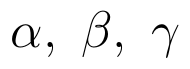with the positive direction of co-ordinate axes, then write the value of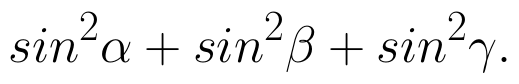(View Answer Video)

Question 3 : Write the distance between the parallel planes 2x - y + 3z = 4 and 2x - y + 3z = 18. (View Answer Video)

Question 4 : Find the vector equation of the line passing through the point (1, 2, 3) and parallel to the planes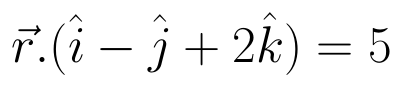and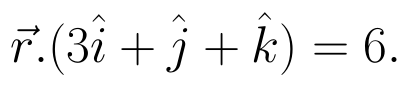(View Answer Video)

Question 5 : Write the vector equation of the plane passing through the point (a, b, c) and parallel to the plane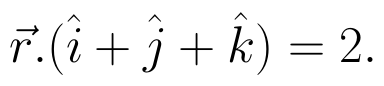(View Answer Video)

### Relations and Functions

Question 1 : If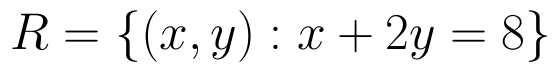is a relation on N, write the range of R. (View Answer Video)

Question 2 : Considergiven by f(x) = 4x + 3. Find the inverse of f. (View Answer Video)

Question 3 : Let A = {1, 2, 3, 4} and let R = {(2, 2), (3, 3), (4, 4), (1, 2)} be a relation on A. Then, R is, (View Answer Video)

Question 4 : If f is the greatest integer function and g is the modulus function . Write the value of g o f(-1/3) – f o g ( -1/3 ). (View Answer Video)

Question 5 :  If f(x) = ax + b and g(x) = cx + d, then f[g(x)] – g[f(x)] is equivalent to, (View Answer Video)

### Application of Integrals

Question 1 : Given that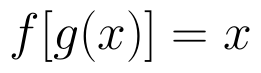, for x = 0 to x = 20. Find f(x) and g(x) such that the area between f(x) and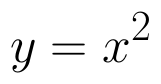from x = 0 to x = 5 be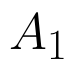and area between g(x) and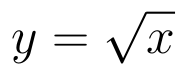from y = 0 to y = 5 be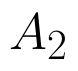. Is? Like functions f and g which work is better, team work or individual work? (View Answer Video)

Question 2 : Find the area enclosed by the parabola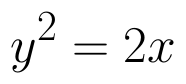and the line x - y = 4. (View Answer Video)

Question 3 : Find the area of smaller region bounded by the ellipse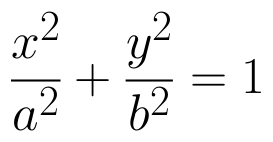and the line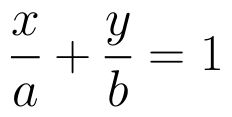. (View Answer Video)

Question 4 : Find the area of the smaller part of the circle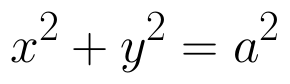cut off by the line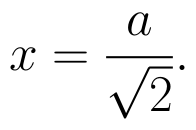(View Answer Video)

Question 5 : Find the area of the region bounded by the curve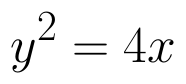and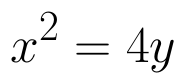. (View Answer Video)

### Inverse Trigonometric Functions

Question 1 : If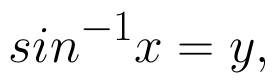then value of y lies between.....? (View Answer Video)

Question 2 : Write the principal value of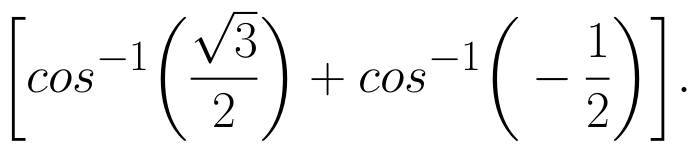(View Answer Video)

Question 3 : Evaluate :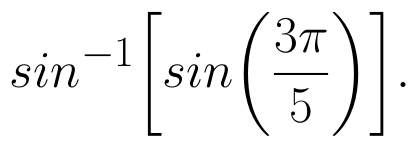(View Answer Video)

Question 4 : Write the principal value of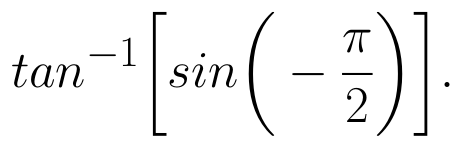(View Answer Video)

Question 5 : Write the principal value of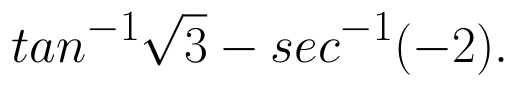(View Answer Video)